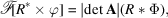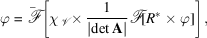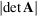International
Tables for
Crystallography
Volume B
Reciprocal space
Edited by U. Shmueli

International Tables for Crystallography (2010). Vol. B, ch. 1.3, p. 47   | 1 | 2 |

## Section 1.3.2.7.1. Shannon's sampling theorem and interpolation formula

G. Bricognea

aGlobal Phasing Ltd, Sheraton House, Suites 14–16, Castle Park, Cambridge CB3 0AX, England, and LURE, Bâtiment 209D, Université Paris-Sud, 91405 Orsay, France

#### 1.3.2.7.1. Shannon's sampling theorem and interpolation formula

| top | pdf |

Letbe such thathas compact support K. Let ϕ be sampled at the nodes of a lattice, yielding the lattice distribution. The Fourier transform of this sampled version of ϕ iswhich is essentially Φ periodized by period lattice, with period matrix A.

Let us assume that Λ is such that the translates of K by different period vectors of Λ are disjoint. Then we may recover Φ fromby masking the contents of a unit cell'of Λ (i.e. a fundamental domain for the action of Λ in) whose boundary does not meet K. Ifis the indicator function of, thenTransforming both sides byyieldsi.e.sinceis the volume V of.

This interpolation formula is traditionally credited to Shannon (1949), although it was discovered much earlier by Whittaker (1915). It shows that ϕ may be recovered from its sample values on(i.e. from) providedis sufficiently fine that no overlap (or aliasing') occurs in the periodization of Φ by the dual lattice Λ. The interpolation kernel is the transform of the normalized indicator function of a unit cell of Λ containing the support K of Φ.

If K is contained in a sphere of radiusand if Λ andare rectangular, the length of each basis vector of Λ must be greater than, and thus the sampling interval must be smaller than. This requirement constitutes the Shannon sampling criterion.

### References

Shannon, C. E. (1949). Communication in the presence of noise. Proc. Inst. Radio Eng. NY, 37, 10–21.
Whittaker, E. T. (1915). On the functions which are represented by the expansions of the interpolation-theory. Proc. R. Soc. (Edinburgh), 35, 181–194.